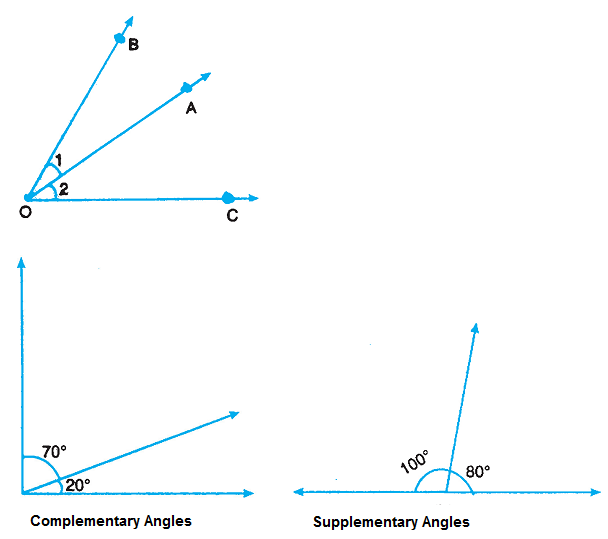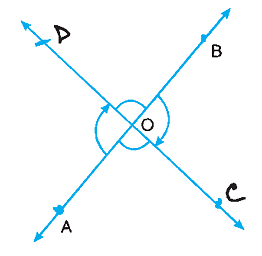# Pair of Angles

Each pair has a common vertex O and a common side OA in between OB and OC. Such a pair of angles is called a pair of adjacent angles.A pair of angles, whose sum is 90°, is called a pair of complementary angles. Each angle is called the complement of the other.

A pair of angles whose sum is 180°, is called a pair of supplementary angles. Each such angle is called the supplement of the other.

### Vertically Opposite Angles

When two intersecting lines AB and CD intersect each other at O, ∠AOC and ∠DOB are angles opposite to each other. These make a pair of vertically opposite angles.∠AOC = ∠DOB

∠AOD = ∠BOC

If two lines intersect each other, the pair of vertically opposite angles are equal.